# Metal alloy

What is the ratio of metals in the alloy that is in the 50 tonnes of steel to 30 kg nickel?

x =  0.06 %
y =  99.94 %
p =  0.0006004

### Step-by-step explanation:

x = 100-y
x = 30/(50•1000)•100
p = 30/(50•1000-30)

x+y = 100
x = 0.06
p = 0.0006

p = 3/4997 ≈ 0.0006
x = 3/50 = 0.06
y = 4997/50 = 99.94

Our linear equations calculator calculates it.
$p=\frac{m\left(Ni\right)}{m\left(Fe\right)}=\frac{30}{50\cdot 1000-30}=\frac{3}{4997}=0.0006004$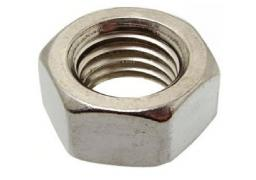Did you find an error or inaccuracy? Feel free to write us. Thank you!Tips to related online calculators
Do you have a linear equation or system of equations and looking for its solution? Or do you have a quadratic equation?

## Related math problems and questions:

• Alloy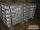The first alloy is a mixture of two metals in the ratio 1:2, the second is a mixture of same metals in the ratio 2:3. At what ratio we have these two alloys put into the furnace to obtain a new metal alloy with ratio 17:27? (All three ratios correspond to
• Coffee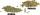Coffee merchant has coffee robusta and arabica species. 1 kg Robusta worth 450 CZK, Arabica 1 kg is 300 CZK more expensive. Calculate how many kilograms of Robusta and Arabica will need to produce 30 kg of the mixture so that the mixture cost is 490 CZK p
• SpiritFrom 55% and 80% spirit we would like to produce 0.2 kg of 60% spirit. How many of them we must use in a solution?
• MixingIf we mix 5 kg of goods of one kind and 3 kg second one, resulting mixture cost 16.50 EUR/kg. If these quantities are mixed in reverse - first three kilograms and 5 kilograms second cost of mixture is 18.50 EUR/kg. What is the price of one kg of goods of
• SaltSeawater containing 5% salt. How many kg of freshwater necessary to pour into 40 kg of seawater so that the salinity fell to 2%?
• SugarsIn what ratio must two sorts of sugar, costing #390 and #315 per kg respectively, be mixed in order to produce a mixture worth #369 per kg?
• CuZn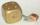Brass is an alloy of copper and zinc. The 10 centimeter brass cube has a weight of 8.6 kg. Copper density is 8930 kg/m3, the zinc density is 7130 kg/m3. Calculate how many kg of copper and zinc a cube contains.
• Alcohol mixtureFrom 55% and 80% alcohol, we have to produce 0.2 kg of 60% alcohol. How many of them do we use in the solution?
• RingThe ring made from gold and copper alloy has a weight of 14.5 g and a volume of 1.03 cm3. How much gold and how much copper it contains? The metal densities are Au 19.3 g/cm³ and Cu 8.94 kg·dm-3
• Mixture of coffee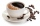They have to prepare a mixture of coffee in packing centres so that 1 kg costs 240 CZK. There are two types of coffee in stock at the price of 220 CZK per 1 kg and 300 CZK per 1 kg. How many kilograms of each species need to be mixed to prepare 50 kg of t
• Acid evaporationHow many kilograms of water do we have to evaporate from 100 kg of 32% acid to make it 80% concentration?
• SweetsWe want to prepare 5 kg of sweets for 150 CZK. We will mix cheaper candy: 1 kg for 120 CZK and more expensive candy: 1 kg per 240 CZK. How much of these two types of candy is necessary to prepare this mixture?
• Sea waterMixing 62 kg of sea water with 84 kg rainwater is created water containing 3.1% salt. How many percent sea water contains salt?
• The aluminumThe aluminum object with a weight of 0.80 kg and a temperature of 250°C was placed in the water with a weight of 1.6 kg and a temperature of 23°C. What is the system temperature after reaching equilibrium? We assume that the heat exchange occurred only be
• CoffeeOne kilogram of cheaper coffee costs CZK 150, one kilogram of more expensive coffee costs CZK 200. We have to prepare a mixture of 35 kg of coffee for 180 CZK. How do we prepare the mixture (how many of which kind of coffee do we need)?
• Apples 2Dried apples contain 15% water. Fresh apples contain 80% water. How many kg of apples we need buy in order to get 3 kg of dried apples?
• Barrel 3Barrel with water weights 118 kg. When we get off 75% of water, it weights 35 kg. How many kg has an empty barrel?